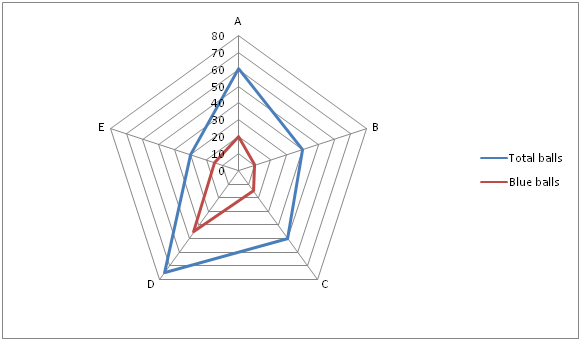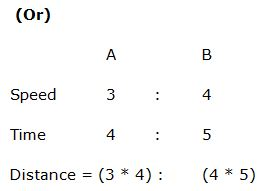# IBPS PO Prelims Quantitative Aptitude Questions 2019 (Day-9)

Dear Aspirants, Our IBPS Guide team is providing new series of Quantitative Aptitude Questions for IBPS PO Prelims 2019 so the aspirants can practice it on a daily basis. These questions are framed by our skilled experts after understanding your needs thoroughly. Aspirants can practice these new series questions daily to familiarize with the exact exam pattern and make your preparation effective.

Check here for IBPS PO Prelims Mock Test 2019

##### Check here for IBPS PO  Mock Test 2019
[WpProQuiz 7027]

1) A man sold an item for Rs. 2000 and incurred a loss. Had he sold the item for Rs. 3600, his gain would have been equal to three times of the amount of loss that he incurred. At what price should he sell the article to gain 20 %?

a) Rs. 2640

b) Rs. 2720

c) Rs. 2960

d) Rs. 2880

e) None of these

2) The sum of lengths of two trains A and B is 640 m. The speed of train A to that of train B is in the ratio of 3 : 4. The time taken by train A and train B to cross an electronic pole is in the ratio of 4 : 5. Find the difference between the length of train A to that of B?

a) 160 m

b) 200 m

c) 180 m

d) 240 m

e) None of these

3) The speed of the boat in still water is 36 km/hr and the speed of stream is 12 km/hr. The time taken by boat to travel from A to B downstream is 3 ½ hours less to travel from B to C upstream. If the distance between A to B is 24 km more than the distance between B to C, then find the distance between A to B?

a) 240 km

b) 200 km

c) 216 km

d) 192 km

e) None of these

4) A and B are 585 km away and both of them start moving towards each other at the same time. After some time when A has travelled 360 km he meets B. What is the ratio of the speed of A to that of B?

a) 11 : 8

b) 5 : 3

c) 7 : 5

d) 8 : 5

e) None of these

5) A mixture of milk and water in a jar contains 32 liters milk and 10 liters water. X liter of milk and X liter of water is added to a new mixture. If 40 % of new mixture is 24 liters, then find the value of X (In liters)?

a) 12

b) 9

c) 16

d) 14

e) None of these

Directions (6 – 10): Study the following information carefully and answer the questions given below.

Following Radar graph shows the total number of balls in 5 different bags and the blue balls in them.6) Find the difference between the average number of balls in the bag A, B and C to the average number of balls other than the blue colour in the bag B, D and E.

a) 25

b) 15

c) 50

d) 150

e) 75

7) Find the ratio of sum of Red color balls in A and D together to the pink color balls in same bags, if the bags have only three color balls and the ratio of pink and red color balls in the bags A and D is 13: 7 and 4: 11 respectively.

a) 17: 18

b) 18: 17

c) 8: 7

d) 7: 8

e) 5: 4

8) The bags have only three color balls. The red color balls in the bags B and C is what percentage of number of blue balls in the bag D, if the grey and red color balls in the bag B and C is in the ratio 3: 2 and 4: 3 respectively?

a) 50%

b) 45%

c) 60%

d) 80%

e) 90%

9) The bags have only three color balls. If the ratio of yellow color balls and pink color balls in the bags is 3: 2, find the average difference between them.

a) 5

b) 4

c) 8

d) 6

e) 9

10) Total number of blue balls in all the bags together is approximately what percentage of total balls in all the bags together?

a) 40%

b) 45%

c) 35%

d) 50%

e) 60%

Direction (1-5) :

Loss = C.P – 2000

Profit = 3600 – C.P

Profit = 3*Loss

3600 – C.P = 3 * [C.P – 2000]

3600 – C.P = 3C.P – 6000

9600 = 4C.P

C.P = 9600/4 = Rs. 2400

SP = 2400 * (120/100) = Rs. 2880

Let the length of train A be x m,

So, the length of train B = 640 – x

The speed of train A to that of train B is in the ratio = 3 : 4 (3y, 4y)

Time = Length of train / Speed

4 = x / 3y

x = 12y -> (1)

(640 – x) / 4y = 5

640 – x = 20y

640 – 12y = 20y

640 = 32y

y = 20

The length of train A = x = 12 * 20 = 240 m

The length of train B = 640 – x = 640 – 240 = 400 m

Required difference = 400 – 240 = 160 m= > 12 : 20 = 3 : 5

8’s = 640

1’s = 80

The difference between the length of train A to that of B

= > 2’s = 160 m

The speed of downstream = 36 + 12 = 48 km/hr

The speed of upstream = 36 – 12 = 24 km/hr

Let the distance between B to C be x km,

The distance between A to B = (x + 24) km

Given,

x / 24 – (x + 24) / 48 = 7/2

(2x – x – 24) / 48 = 7/2

x – 24 = 168

x = 192

The distance between A to B = x + 24 = 192 + 24 = 216 km

Distance travelled by A when he met = 360 km

Distance travelled by B when he met = 585 – 360 = 225 km

The ratio of the speed of A to that of B

= > 360 : 225

= > 8 : 5

(40/100) * New mixture = 24

New mixture = 24 * (5/2) = 60 liters

Given,

32 + X + 10 + X = 60

42 + 2X = 60

2X = 18

X = 9

Direction (6-10) :

Number of balls other than the blue colour in the bag,

B = 40 – 10 = 30

D = 75 – 45 = 30

E = 30 – 15 = 15

The average = (30 + 30 + 15)/3 = 25

Average number of balls in the bag A, B and C =(60 + 40 + 50)/3 = 50

Required difference = 50 – 25 = 25

Bag A:

Red + Pink = 60 – 20 = 40

Red = (40/20) * 7 = 14

Pink = (40/20) * 13 = 26

Bag D:

Red + Pink = 75 – 45 = 30

Red = (30/15) * 11 = 22

Pink = (30/15) * 4 = 8

Required ratio = (14 + 22): (26 + 8)

= 18: 17

Number of balls other than the blue colour in the bag,

B = 40 – 10 = 30

Red balls in B = (30/5) * 2 = 12

C = 50 – 15 = 35

Red balls in C = (35/7) * 3 = 15

Required percentage = [(12 + 15)/45] * 100 = 60%

Number of balls other than the blue colour in the bag,

A = 60 – 20 = 40

B = 40 – 10 = 30

C = 50 – 15 = 35

D = 75 – 45 = 30

E = 30 – 15 = 15

The sum = 40 + 30 + 35 + 30 + 15 = 150

The ratio of yellow color balls and pink color balls in the bags = 3: 2

Yellow color balls = (150/5) * 3 = 90

Pink color balls = (150/5) * 2 = 60

Required difference = (90/5)- (60/5) = 30/5 = 6